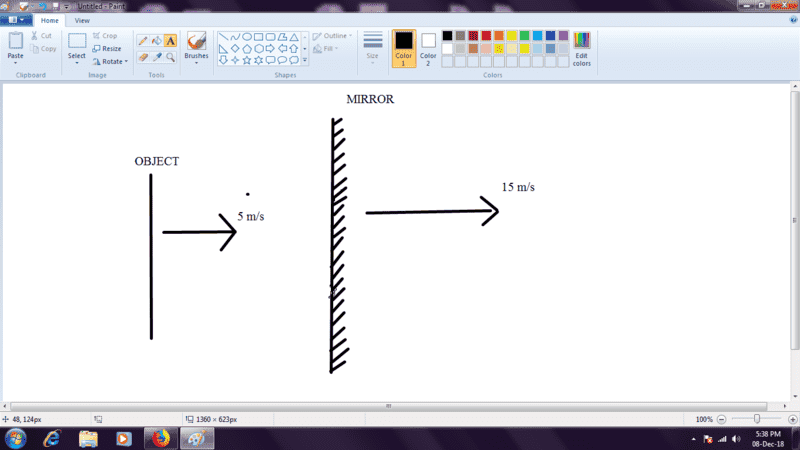# Velocity of image in plane mirror

## Homework StatementA mirror is moving to the right with a velocity 15 m/s, and an object is moving to the right with a speed of 5m/s. All speeds are wrt ground. Find the velocity of image wrt ground.

## The Attempt at a Solution

I present to you two probable solutions:
1) Using formula: VIMAGE=2VMIRROR-VOBJECT
Now, its a mere substitution problem. Using right as positive direction, we get the required velocity as 25m/s to the right.
2) This is a controversial one. I thought about this as i like to explore all the different correct of incorrect methods of a problem.
the above problem is equivalent to: the mirror is moving to the right with a velocity of 10m/s and the object is at rest. Now, substituting in the formula used in the previous method, VIMAGE=20m/s to the right.

Now, my book says that that the correct answer is 10m/s, but its not matching with any of my answers. Please check it and let me know why both are incorrect.

#### Attachments

Homework Helper
Gold Member
You could try making two scale drawings, one showing the starting position of the object, mirror and image, and another showing their positions after say 1 second. Figure out how far the image moved in that time.

Yeah, i'll try by that method.

Homework Helper
Gold Member
PS: Book answers are sometimes wrong.

••